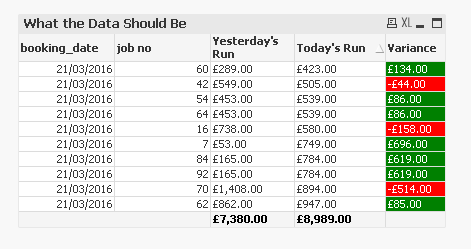# QlikView Scripting

Discussion Board for collaboration on QlikView Scripting.

Announcements

#### Breathe easy -- you now have more time to plan your next steps with Qlik! QlikView 11.2 Extended Support is now valid through December 31, 2020. Click here for more information.Not applicable

## Exclude rows based on set analysis value

I have a sample list of jobs with a spend value per job based on a previous days snapshot and today's snapshot. I need to exclude all rows of data which have 0 in the variance column

Yesterdays Amount uses the following Set analysis - sum({<rundate ={"\$(vPreviousRunDate)"}>} total_amount)

Today's Amount uses the following Set analysis - sum({<rundate ={"\$(vCurrentRunDate)"}>} total_amount)

Variance uses the following set analysis -  sum({<rundate ={"\$(vCurrentRunDate)"}>} total_amount) - sum({<rundate ={"\$(vPreviousRunDate)"}>} total_amount)

Booking DateJob NoYesterday's AmountTodays AmountVariance
01/03/201611141347576656
02/03/20162631963190
03/03/2016314887474910138
04/03/20164752975290
05/03/201651119637347462
06/03/20166216621660
07/03/2016721179201197
08/03/20168250825080
09/03/2016968546548306
10/03/2016101477327114502
11/03/2016111230495962708
12/03/201612719271920
13/03/2016138291460-631
14/03/201614381038100
15/03/2016151226856411704
16/03/2016161214423109834
17/03/2016176066060
18/03/201618246424640

Any help would be appreciated.

6 Replies
Esteemed Contributor III

## Re: Exclude rows based on set analysis valueNot applicable

## Re: Exclude rows based on set analysis value

how to I incorporate this into my set analysis which is used in the variance columnMVP & Luminary

## Re: Exclude rows based on set analysis value

Try using some helper variables:

Variables:

vAmountPrev: sum({<rundate ={'\$(vPreviousRunDate)'}>} total_amount)

vAmountCur: sum({<rundate ={'\$(vCurrentRunDate)'}>} total_amount)

vVariance: rangesum(\$(vAmountCur),-\$(vAmountPrev))

Chart expressions:

=sum({<rundate ={"\$(vPreviousRunDate)"}, [Job No]={"=\$(vVariance)<>0"}>} total_amount)

=sum({<rundate ={"\$(vCurrentRunDate)"}, [Job No]={"=\$(vVariance)<>0"}>} total_amount)

=\$(vVariance)

talk is cheap, supply exceeds demandNot applicable

## Re: Exclude rows based on set analysis value

I couldn't get your solution to work properly. the variables seem to have calculated the variance between the total data set. I need to compare the total amounts for the individual job between the 2 different rundatesMVP

## Re: Exclude rows based on set analysis value

Like this?Expressions:

1)

If(Sum({<rundate ={"\$(vCurrentRunDate)"}>} total_amount) - Sum({<rundate ={"\$(vPreviousRunDate)"}>} total_amount) <> 0,

Sum({<rundate ={"\$(vPreviousRunDate)"}>} total_amount))

2)

If(Sum({<rundate ={"\$(vCurrentRunDate)"}>} total_amount) - Sum({<rundate ={"\$(vPreviousRunDate)"}>} total_amount) <> 0,

Sum({<rundate ={"\$(vCurrentRunDate)"}>} total_amount))

3)

=sum({<rundate ={"\$(vCurrentRunDate)"}>} total_amount) - sum({<rundate ={"\$(vPreviousRunDate)"}>} total_amount)MVP & Luminary

## Re: Exclude rows based on set analysis value

Don't put an = character in front of the expression in the variable if I didn't. And change the double quotes in the variables to single quotes:

Variables:

vAmountPrev: sum({<rundate ={'\$(vPreviousRunDate)'}>} total_amount)

vAmountCur: sum({<rundate ={'\$(vCurrentRunDate)'}>} total_amount)

vVariance: rangesum(\$(vAmountCur),-\$(vAmountPrev))

talk is cheap, supply exceeds demand
Community Browser